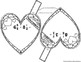# Add and Subtract Mixed Numbers Valentine's Day Heart PennantsSubject
Resource Type
File Type

PDF

(3 MB|20 pages)
Standards
Also included in:
1. Students add, multiply and divide mixed numbers in these math activities that double as Valentine's Day classroom decor. Once a pennant is complete, it can be hung along a string in your classroom to show the world that, "Hey, we know mixed numbers!"Included are 60 pennants, 4 additional pennants wi
\$8.00
\$5.50
Save \$2.50
• Product Description
• Standards

Students add and subtract mixed numbers with unlike denominators in this math activity that doubles as Valentine's Day classroom decor. Once a pennant is complete, it can be hung along a string in your classroom to show the world that, "Hey, we know how to add and subtract mixed numbers!"

Problems vary in difficulty making differentiation easy. The first 15 pennants ask students to add or subtract mixed numbers where one denominator is already the LCD (ex: 4 7/10 + 9 2/5). The last 15 pennants ask students to add or subtract mixed numbers where they have to find the common denominator between the two fractions (ex: 8 1/3 + 9 4/7).

Included are 30 pennants, 2 additional pennants without fraction problems (for you to write your own), an optional student answer sheet and an answer key.

Each group of students can be given a set of pennants, scissors and either glue, tape or a stapler. Students can be in charge of cutting out the pennants. Groups can compete to see which group can complete the most pennants or your entire class can add pennants to one string as they complete each one. You may also choose to have your students color their pennants to add to your classroom decor.

See more Valentine's Day math activities.

You may also like:

Pi Day Pennant

Math Pennants Bundle

Add and subtract fractions with unlike denominators (including mixed numbers) by replacing given fractions with equivalent fractions in such a way as to produce an equivalent sum or difference of fractions with like denominators. For example, 2/3 + 5/4 = 8/12 + 15/12 = 23/12. (In general, 𝘢/𝘣 + 𝘤/𝘥 = (𝘢𝘥 + 𝘣𝘤)/𝘣𝘥.)
Total Pages
20 pages
Included
Teaching Duration
N/A
Report this Resource to TpT
Reported resources will be reviewed by our team. Report this resource to let us know if this resource violates TpT’s content guidelines.